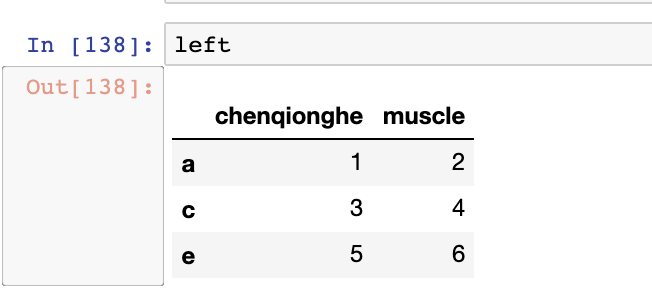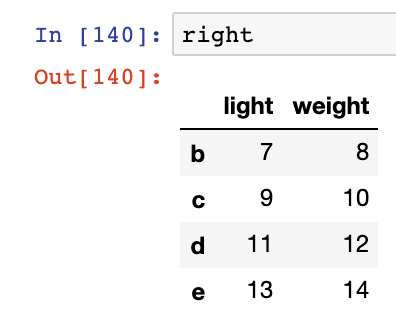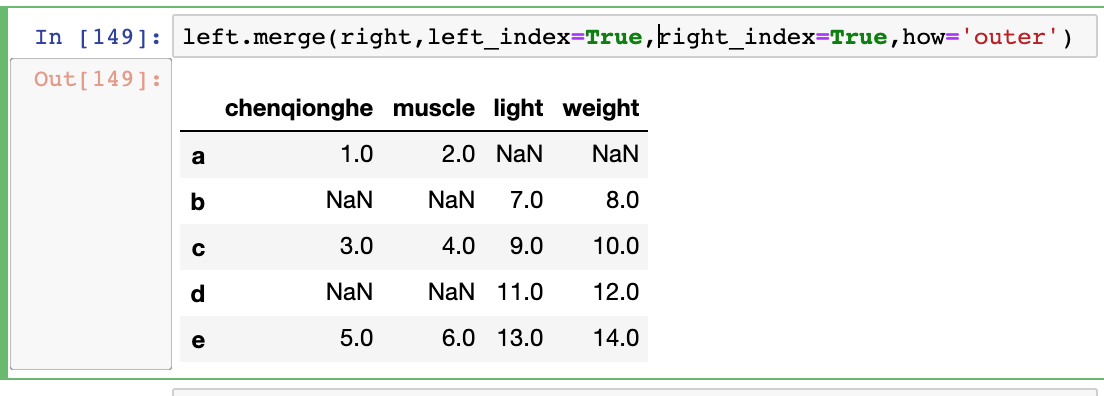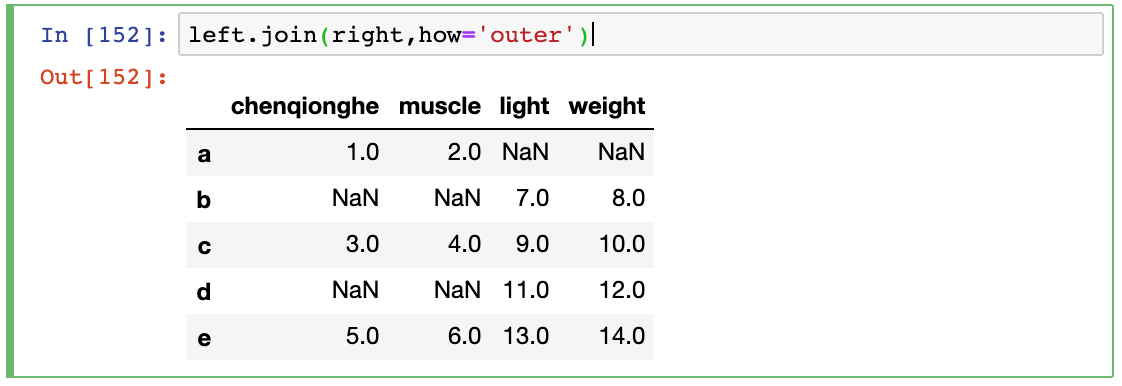# Pandas中merge和join的区别

2020/01/23 22:07

## 示例

left=pd.DataFrame([
[1,2],[3,4],[5,6]
],
index=['a','c','e'],
columns=['chenqionghe','muscle']
)定义一个right的DataFrame

right=pd.DataFrame([
[7,8],[9,10],[11,12],[13,14],
],
index=['b','c','d','e'],
columns=['light','weight']
)left.merge(right,left_index=True,right_index=True,how='outer')left.join(right,how='outer')## merge的参数

• left：参与合并的左侧DataFrame
• right：参与合并的右侧DataFrame
• how：inner、outer、left、right其中之一
• left_index：将左侧的行索引用作其连接键的列
• right_index：类似于left_index
• sort：根据连接键对合并后的数据进行排序，默认为True。有时在处理大数据集时，禁用该选项可获得更好的性能
• suffixes：字符串值元组，用于追加到重叠列名，默认为（'_x','_y'）
• copy：设置为False，可以在某些我死情况下避免将数据复制到结果数据结构中，默认总是复制

0
0 收藏

### 作者的其它热门文章0 评论
0 收藏
0